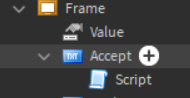# Script not working correctly

Sorry if this is vague but I am extremely delirious right now.

This script just isn’t working and I don’t know why.

``````
local Id = math.random(1, 10000000)
repeat
if Check then
Id = math.random(1, 10000000)
else
end
wait(0.1)
until Check == false
script.Parent.Parent.Value.Changed:Connect(function()
print("hey")
if script.Parent.Parent.Value.Value ~= nil then
print("yo")
end
``````

No output, for some reason.This is the script

Thanks.

Haven’t looked into the script, but I can see a ServerScript inside a GUI which is usually ran by the client. Have you tried a LocalScript for things not ran by the server?

``````local Storage = game:GetService("ReplicatedStorage")
local Id = math.random(1, 10000000)

repeat
if Check then
Id = math.random(1, 10000000)
else
end
until not Check

script.Parent.Parent.Value.Changed:Connect(function()
if script.Parent.Parent.Value.Value ~= nil then